# The Convex Hull Problem

### February 2, 2011 12:00

If you are unfamiliar with the convex hull problem, take a gander.

Recently I was tasked with writing a divide-and-conquer convex hull algorithm. Despite there being several algorithms that already exist, I decided to take the task into my own hands and develop my own algorithm for solving the problem. After some effort on my own part, I was able to develop an algorithm that solves the convex hull problem in Big-O nlogn time in the average case. In very rare occasions, the algorithm can degenerate into Big-O n^2 time (when points are distributed evenly in a circle), but for nearly all other situations, the algorithm solves large data sets in a very short amount of time. I ran a series of tests on my small netbook with a single 2 GHz processor and was able to generate a solution to a data set of 1,000,000 points in just under two seconds. So, let's get to it, shall we?

Let's take a data set of 35 points, represented in the image below: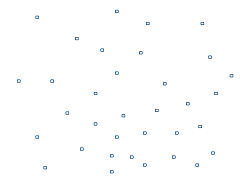The points don't need to be ordered in any way. If they are ordered in any way, it will have zero effect on the algorithm run time. The only things that affect the algorithm's run time are the number of points and their distribution. No other factors affect the algorithm's efficiency. We will loop through all the points and find the following:

1. The point with the greatest X-value
2. The point with the smallest X-value
3. The point with the greatest Y-value
4. The point with the smallest Y-value
This will leave us with four points: From these four, make four line segments, creating a diamond shape: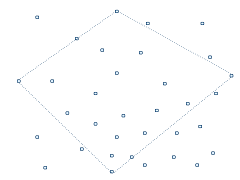These four points will always be a part of the convex hull. We then enter a recursive function on each of these four line segments. For each segment, we find which point is furthest from the segment. We create a segment connecting the three points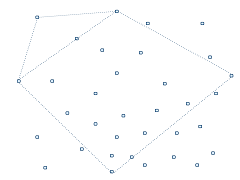Notice how all of these lines are still dotted. This means that we have not determined whether these lines will actually be part of the convex hull. These lines will be solidified as we reach the end of each recursive function. Since there are no points points on the outside of either of these line segments, these lines are solidifed and we have determined that these line segments are part of the ultimate convex hull.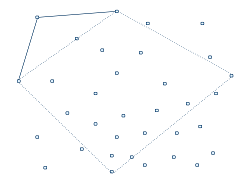Now we move onto the next line segment. We find the outer point, create line segments, and end the recursive calls.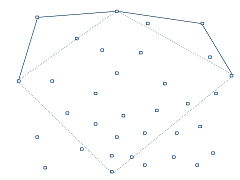The next segment acts a bit differently. First, we find the point furthest from the segment.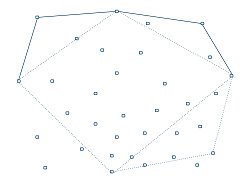On the first line, there is no point futher out, but on the second segment, there is still a point outside of the segment. So, we enter deeper into the recursion to continue finding points outside of the current line segments.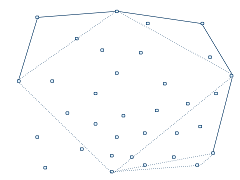Fortunately for us, there are no more points outside of either of these segments, so we have solidifed one more side. We move on to the next side on the bottom left, repeat the same process, and end up with the following.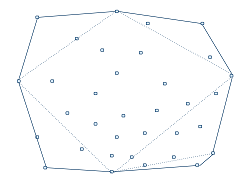And there we have it! We keep only the solid lines and we have created our convex hull.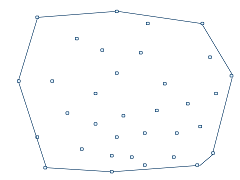The Pseudo-code for the algorithm might look something like this:

``````Get the points with max x-value, max y-value, min x-value and min y-value (These points will always be on the resulting hull)
With each line segment defined by p1 and p2:
Find the point furthest away on the outside of this line segment
if there is no point, end the calls on this curve
if there is, add the point to the list of points on the outer hull and call this method again with segments p1 -> point and point -> p2``````
Here's the algorithm written in C#

Enjoy!

## Got something to say? Tell me!

Name*

Homepage

Comment*

Feb.2.2011
23:57

J

What happens when the point with the smallest x-coordinate is also the point with the smallest y-coordinate?

Feb.3.2011
00:14

J

I am also unsure of how this algorithm is nlogn...could you explain? I don't see a sort or a logarithmic component of any kind. The recursive statements don't get any smaller...it looks like you pass in and iterate over the entire list of points in each recursion. It seems like O(hn) to me, where h is...I duno...the number of points on the hull, I think.

Feb.3.2011
00:15

J

It seems my first comment never made it...which was "what happens when the point with the smallest x-coordinate is also the point with the smallest y-coordinate (or largest and largest)?"

Feb.3.2011
08:15

Andrew W

Thanks for the comments. You raise a valid point, and I even began to question my own algorithm. I'm still unsure as to whether or not the algorithm in the current state really is nlogn, but I knew it could be.

I went back and made some changes to the algorithm which I'm sure is now nlogn. The biggest change is that instead of looking at all the points during every recursive check, it only looks at the points that were deemed outside of the segment on the previous recursive check. Putting this little piece greatly improved the algorithm and on my quick tests, seemed to drop the worst-case scenario of n^2 and always be nlogn. I'll do some more checks later today and post the results.

Feb.3.2011
08:20

Andrew W

With respect to when you end up with 3 points instead of 4 at the first step, you simply end up with 3 points. In reality, I originally had it where you just looked for the points at the smallest and greatest X value and started from there, but I picked up a little on the constant efficiency by grabbing all 4 values in that first loop instead of 2.

Feb.3.2011
20:20

J

I think the worst case, even with that revision, is still O(n^2). In this case, the worst case is no longer a circle (which I agree is O(nlogn)). The worst case would be if all remaining points (after you find the initial four) lie along a line parallel to one of the hull sides, i.e. if the distance of all remaining points is the same. So imagine a diamond with a line (outside the diamond) parallel to one side of the diamond.

In this case, examining the points deemed outside the segment on the previous recursive check would only decrease the size of the array of points by 1 each time.

The first three recursive statements end in one step, so they are O(n) each; the last recursive statement, however, would check n points; then, it would find that n-1 (n-4, actually, but n-1 for illustration purposes) points remain outside the line; it would pass this array into the next recursive call, which then finds that n-2 points remain outside, then n-3, etc.

Thus, the algorithm performs n+(n-1)+(n-2)+(n-3)+...+(n-k) checks. This turns out to be the sum from 1 to n, which is n(1+n)/2, which simplifies into n^2.

In more rigorous terms, the recurrence relation for that recursive statement is:

T(n) = n + T(n-1) + 1; T(1) = 1
T(n) = n + [ (n-1) + T(n-2) + 1] + 1
T(n) = n + (n-1) + (n-2) + (n-3) + ... + (n-k) + k
when k = n,
T(n) = n + (n-1) + (n-2) + (n-3) + ... + (1) + n= n(1+n)/2 + n

and that simplifies into n^2. It's been a while since algorithms...so I could be wrong. Sorry I'm being "that guy"...but this is what happens when you're a bored ex-CS major.

Feb.3.2011
20:22

J

Does the denominator variable in distanceFromPointToSegment become zero if you only find three initial points (i.e. p1=p2)? Does that cause a divide by zero error in the next line?

Feb.3.2011
21:43

Andrew W

No worries at all about being "that guy". I really appreciate all the comments - you can be sure that everything you've mentioned I've taken seriously.

I'd first like to address your comment about the algorithm degenerating down to n^2 when you have a parallel line along one of the 4 edges once you've created the diamond shape. Let me walk you through what would happen:

Assuming we have a line of 50 points along the top right line running parallel. The algorithm would loop through all of these points. BUT, since they all have the same distance from the segment, it would grab the first point and move to the next step. The left side of that Inner loop would have no outer points so it would exit. The right side would no longer be parallel to that list of points. Instead, it would see the last point in that line of points as the furthest. It would grab that point and move on into the recursion. At this point, there is nothing along the outside of the segment, so the recursion ends. It would not loop over the points again.

This presents an interesting problem. It didn't grab all of the points. But, it still outputted the correct line segments. Is this a problem? I guess it depends on the desired output. If you want every point that the hull would use, my algorithm fails. (But does it? Sure, the line runs along all the points, but the hull doesn't "wrap" around any of those points, so saying they aren't included in the outer hull may be justified) If the output you want is solely the shape of the hull, my algorithm still works. If the output you want is the actual points included in the outer hull, my results may be argued incomplete.

Regarding your comment about the denominator being zero never happens, but is presenting yet another corner case I hadn't considered =). I'll look into it further.

Feb.3.2011
21:48

J

I hate being wrong.

Feb.3.2011
21:57

Andrew W

No worries - happens to the best of us. It's good that you're helping me get all the corner cases worked out. My next goal is to take this algorithm up to the third dimension maintaining time-efficiency and ultimately up to 'n' dimensions, so getting all the bugs worked out while I'm still down here in 2d land is helping immensely.

Feb.3.2011
23:29

J

Hm, so actually the case of perpetually unbalanced sides does exist...it's not as I described earlier -- it's the far more ridiculous case in which the points lie along a quarter-circle, but only along midpoints biased on one side of the arc-midpoint (if that makes any sense at all). So if you're traveling from pi/2 to 0 along an arc, having points at pi/4, then pi/8, then pi/16, then pi/32, etc. would cause the algorithm to enter an n + (n-1) + (n-2) + ... + 1 recurrence relation. But that sort of data is too highly organized to be realistic.